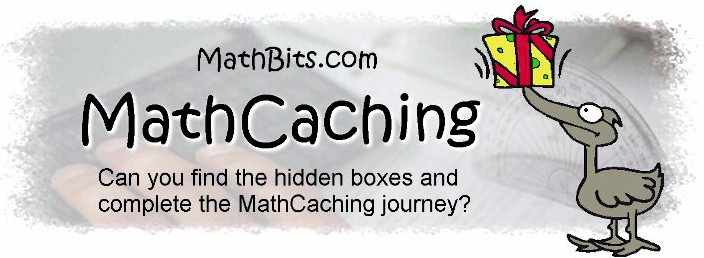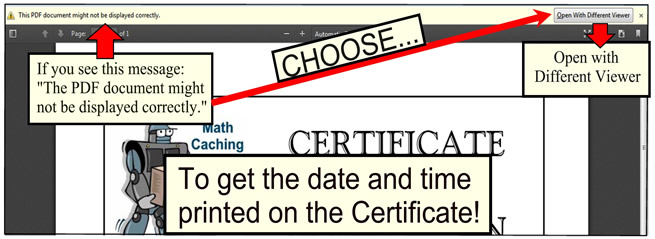"Geocaching" (pronounced "geo cashing") is the sport of using GPS systems to locate hidden boxes at different geographical locations across the country. Directions and clues are left in the boxes to aid in the journey.

In the spirit of geocaching, we have created activities called "MathCaching" which use the internet to find hidden boxes to reveal clues to the continuation of the games. Your success at "MathCaching" is dependent upon your skills at solving mathematical problems.

The MathCaching games are subject area dependent. There are free versions of MathCaching for Basic Math Skills ("BasicCaching"), Algebra ("AlgeCaching"), Geometry ("GeoCaching"), Algebra2 (Alge2Caching), Trig ("TrigCaching"), PreCalculus ("PreCalcuCaching"), Calculus ("CalcuCaching") and TI-84+ Caching.

The MathCaching screens are deliberately "large" in print so that they can be projected for classroom use. A teacher answer key (including the URL addresses of all of the boxes) for each free game is available upon request for teachers from a school district email address.

 Note to Educators: The free MathCaching games at MathBits cover topics that occur throughout each of the respective courses and are best used for review at the end of the year, or for warm-up at the beginning of the year for the following course. Each of the MathBits' paid subscription areas (JrMathBits.com, AlgebraBits.com, GeometryBits.com, Algebra2Bits.com) includes MathCaching games for each "topic" area, referred to as the "MathCaching Topic Series" which are played in the same fashion. The CERTIFICATE found at the end of each Caching Game will carry a date and time stamp WHEN PRINTED. If your browser is FireFox, be sure to follow the directions below:Use the Certificate, along with the work shown on the student worksheet, as evidence of a student's completion of the game.

To Play the Open (Free) Versions of MathCaching:

1. Download the MathCaching game's matching worksheet to record your work and your answers.

2. Go to the "START the GAME" internet URL address for your chosen area.

3. Solve the math problem(s) at that location.

4. Follow the directions (based upon your answers) to find the address of the next hidden internet box.

5. Into your browser, TYPE the address of the next hidden box, using your answer to fill in the missing portion of the URL address.

6. When you find the final hidden box, a certificate is available stating that all of the hidden boxes have been discovered. Students may want to print the certificate to share their success with their math teacher, or teachers may request the certificate (along with the completed worksheet) to verify that the activity was completed.

7. Good luck with your MathCaching journey!!

Please note that URL addresses are case sensitive!!

 Levels START the GAME - URL Address Basic Math Worksheet http://mathbits.com/caching/basicopencache1.html Topics: computations, fractions, ratios, percents, order of operations, signed numbers, exponents, word problem translations, measurement conversions, sum of angles in a triangle, mean, median, expressions, probability, patterns. PreAlgebra Worksheet http://mathbits.com/caching/prealgcache1.html Topics: order of operations, evaluating expressions, signed numbers, slopes, equations of lines, charts of values, Pythagorean Theorem, solving equations, polynomials, inequalities, mean and median of data, perimeter, area, volume, perfect square radicals, simplifiying numerical fractions, absolute value, greatest common factor. Algebra 1 Worksheet http://mathbits.com/caching/opencache1.html Topics: order of operations, equation solving, integer and consecutive integer word problems, quadratic equaions, systems, factoring, area, exponents, box plots, slopes, functions, exponetials. Geometry Worksheet http://mathbits.com/caching/geoopencache1.html Topics: angles, reasoning, parallel and perpendicular lines, triangles, congruent triangles and proof, quadrilaterals, area and volume, polygons, similarity, circles, transformations. Algebra2 Worksheet http://mathbits.com/caching/alg2opencache1.html Topics: absolute value equations and inequalities; radical equations; functions: domains, composition, inverse; logarithms; exponentials; rationalizing denominators; quadratics: graphing, discriminant, complete the square; complex fractions; asymptotes; rational equations; sequences and series, Binomial Theorem; probability. Trigonometry Worksheet http://mathbits.com/caching/trigopencache1.html Topics: right triangle trig, angle relationships, conversion between radians and degrees, reference angles, exact values, area of triangle, Law of Sines, Law of Cosines, amplitude, frequency, period, vertical shift, phase shift, inverse trig functions, trig identities, trig equations. PreCalculus Worksheet http://mathbits.com/caching/precalccache1.html Topics: algebra of functions, inverse functions, composition of functions, difference quotient, evaluation of functions, equations of circles-parabolas-ellipses, complex numbers, product of roots, sum of roots, remainders, summations, arithmetic and geometric sequences, trigonometry, rate of growth and decay, systems and linear programming, and asymptotes for rational functions. Differential Calculus Worksheet http://mathbits.com/caching/CalcCacheDiff1.html Topics: functions (domain, odd/even, composition, inverse), limits, continuity, definition of derivative, derivatives, linearization, differentiable functions, relative maxima/minima, intervals of increasing/decreasing, inflection point, intervals of concavity, applied derivatives (first and second derivative test), related rates, trigonometric derivatives including inverse trig functions, derivative of natural logarithmic function. Questions are both open ended and 5-choice AP-style multiple choice. Integral Calculus coming .... TI-84+ Graphing Calculator Intro. Level Worksheet http://mathbits.com/caching/TI84Cache1.html This game is designed at the Introductory Level of calculator use, and is appropriate for beginners who are just discovering the calculator, or as a refresher for forgotten skills. Topics: basic calculator information, basic operational skills, basic graphing skills, statistics and probability, and dealing with errors. The mathematical level is designed for basic algebra skills. Questions are designed to be applicable to the TI-83+ and TI-84+ in both "MathPrint" and "Classic" modes. TI-84+ Graphing Calculator Advanced Level coming .... Holiday Caching (seasonal only) http://mathbits.com/caching/holiday/holidaycaching.html Level 1 Topics: ratio, percentages, area, perimeter, circumference, Pythagorean Theorem, logical reasoning. Word problems. Level 2 Topics: algebraic expressions, area, perimeter, mean, solving linear equations, circumference, quadratic equations, exponents, order of operations, logical reasoning. Word problems. Level 3 Topics: types of angles and triangles, Pythagorean Theorem, parallel lines and angles, congruent triangles, quadratic equations,logical reasoning. Word problems. Level 4 Topics: functions, basic trigonometry, exponential expressions, linear quadratic systems, inequalities, polynomials, equations of circles, area of circles, logical reasoning. Word problems.